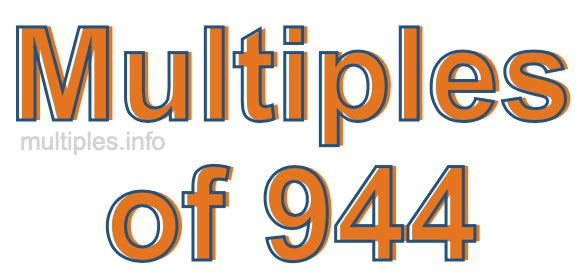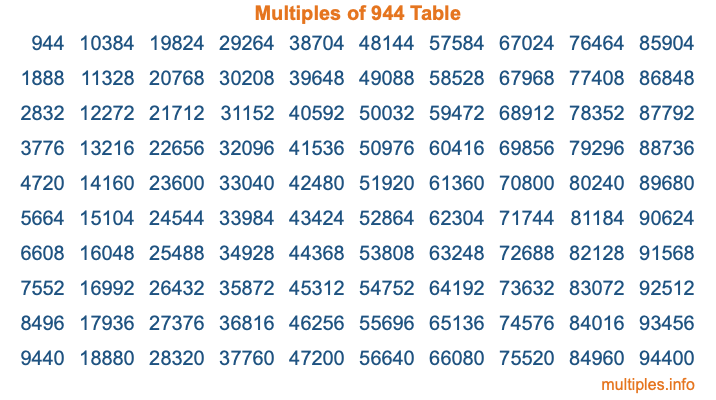Multiples of 944Welcome to the Multiples of 944 page. Here we will first teach you everything you will ever need to know about the multiples of 944, and then give you a study guide summary of everything we taught you to make sure you remember it all. Use this page to look up facts and learn information about the multiples of 944. This page will make you a multiples of nine hundred forty-four expert!

Definition of Multiples of 944
Multiples of 944 are all the numbers that when divided by 944 equal an integer. Each of the multiples of 944 are called a multiple. A multiple of 944 is created by multiplying 944 by an integer.

Therefore, to create a list of multiples of 944, you start with 1 multiplied by 944, then 2 multiplied by 944, then 3 multiplied by 944, and so on for as long as you want. Thus, the list of the first five multiples of 944 is 944, 1888, 2832, 3776, and 4720. To see a larger list of multiples of 944, see the printable image of Multiples of 944 further down on this page. We also have a category where you can choose any nth multiple of 944.

Multiples of 944 Checker
The Multiples of 944 Checker below checks to see if any number of your choice is a multiple of 944. In other words, it checks to see if there is any number (integer) that when multiplied by 944 will equal your number. To do that, we divide your number by 944. If the the quotient is an integer, then your number is a multiple of 944.

Is  a multiple of 944?

Least Common Multiple of 944 and ...
A Least Common Multiple (LCM) is the lowest multiple that two or more numbers have in common. This is also called the smallest common multiple or lowest common multiple and is useful to know when you are adding our subtracting fractions. Enter one or more numbers below (944 is already entered) to find the LCM.

Check out our LCM Calculator if you need more details about the Least Common Multiple or if you need the LCM for different numbers for adding and subtraction fractions.

nth Multiple of 944
As we stated above, 944 is the first multiple of 944, 1888 is the second multiple of 944, 2832 is the third multiple of 944, and so on. Enter a number below to find the nth multiple of 944.

th multiple of 944

Multiples of 944 vs Factors of 944
944 is a multiple of 944 and a factor of 944, but that is where the similarities end. All postive multiples of 944 are 944 or greater than 944. All positive factors of 944 are 944 or less than 944.

Below is the beginning list of multiples of 944 and the factors of 944 so you can compare:

Multiples of 944: 944, 1888, 2832, 3776, 4720, etc.

Factors of 944: 1, 2, 4, 8, 16, 59, 118, 236, 472, 944

As you can see, the multiples of 944 are all the numbers that you can divide by 944 to get a whole number. The factors of 944, on the other hand, are all the whole numbers that you can multiply by another whole number to get 944.

It's also interesting to note that if a number (x) is a factor of 944, then 944 will also be a multiple of that number (x).

Multiples of 944 vs Divisors of 944
The divisors of 944 are all the integers that 944 can be divided by evenly. Below is a list of the divisors of 944.

Divisors of 944: 1, 2, 4, 8, 16, 59, 118, 236, 472, 944

The interesting thing to note here is that if you take any multiple of 944 and divide it by a divisor of 944, you will see that the quotient is an integer.

Multiples of 944 Table
Below is an image of the first 100 multiples of 944 in a table. The table is in chronological order, column by column. The first column has the first ten multiples of 944, the second column has the next ten multiples of 944, and so on.The Multiples of 944 Table is also referred to as the 944 Times Table or Times Table of 944. You are welcome to print out our table for your studies.

Negative Multiples of 944
Although not often discussed or needed in math, it is worth mentioning that you can make a list of negative multiples of 944 by multiplying 944 by -1, then by -2, then by -3, and so on, to get the following list of negative multiples of 944:

-944, -1888, -2832, -3776, -4720, etc.

Multiples of 944 Summary
Below is a summary of important Multiples of 944 facts that we have discussed on this page. To retain the knowledge on this page, we recommend that you read through the summary and explain to yourself or a study partner why they hold true.

There are an infinite number of multiples of 944.

A multiple of 944 divided by 944 will equal a whole number.

944 divided by a factor of 944 equals a divisor of 944.

The nth multiple of 944 is n times 944.

The largest factor of 944 is equal to the first positive multiple of 944.

944 is a multiple of every factor of 944.

944 is a multiple of 944.

A multiple of 944 divided by a divisor of 944 equals an integer.

944 divided by a divisor of 944 equals a factor of 944.

Any integer times 944 will equal a multiple of 944.

Multiples of a Number
Here you can get the multiples of another number, all with the same attention to detail as we did for multiples of 944 on this page.

Multiples of
Multiples of 945
Did you find our page about multiples of nine hundred forty-four educational? Do you want more knowledge? Check out the multiples of the next number on our list!